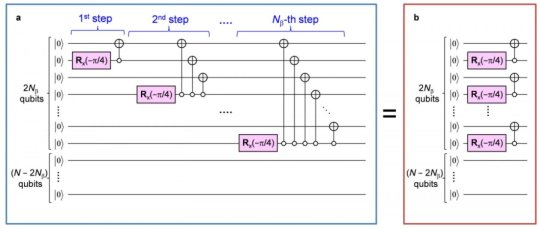# Tech: Quantum chemical calculations on quantum computers — (Report)

0
948Quantum computing and quantum information processing technology have attracted attention in recently emerging fields. Among many important and fundamental issues in nowadays science, solving Schroedinger Equation (SE) of atoms and molecules is one of the ultimate goals in chemistry, physics and their related fields. SE is “First Principle” of non-relativistic quantum mechanics, whose solutions termed wave-functions can afford any information of electrons within atoms and molecules, predicting their physicochemical properties and chemical reactions. Researchers from Osaka City University (OCU) in Japan, Dr. K. Sugisaki, Profs. K. Sato and T. Takui and coworkers have found a quantum algorithm enabling us to perform full configuration interaction (Full-CI) calculations for any open shell molecules without exponential/combinatorial explosion. Full-CI gives the exact numerical solutions of SE, which are one of the intractable problems with any supercomputers. The implementation of such a quantum algorithm contributes to the acceleration of implementing practical quantum computers.

The paper has been published on December 13th, 2018 in the first issue of Open Access Journal Chemical Physics Letters X.

They said, “As Dirac claimed in 1929 when quantum mechanics was established, the exact application of mathematical theories to solve SE leads to equations too complicated to be soluble. In fact, the number of variables to be determined in the Full-CI method grows exponentially against the system size, and it easily runs into astronomical figures such as exponential explosion. For example, the dimension of the Full-CI calculation for benzene molecule C6H6, in which only 42 electrons are involved, amounts to 1044, which are impossible to be dealt with any supercomputers.”

According to the OCU research group, quantum computers can date back to a Feynman’s suggestion in 1982 that the quantum mechanics can be simulated by a computer itself built of quantum mechanical elements which obey quantum mechanical laws. After more than 20 years later, Prof. Aspuru-Guzik, Harvard Univ. (Toronto Univ. since 2018) and coworkers proposed a quantum algorithm capable of calculating the energies of atoms and molecules not exponentially but polynomially against the number of the variables of the systems, making a breakthrough in the field of quantum chemistry on quantum computers.

When Aspuru’s quantum algorithm is applied to the Full-CI calculations on quantum computers, good approximate wave-functions close to the exact wave-functions of SE under study are required, otherwise bad wave-functions need an extreme number of steps of repeated calculations to reach the exact ones, hampering the advantages of quantum computing. This problem becomes extremely serious for any open shell systems, which have many unpaired electrons not participating in chemical bonding. The OCU researchers have tackled this problem, one of the most intractable issues in quantum science, and made a breakthrough in implementing a quantum algorithm generating particular wave-functions termed configuration state functions in polynomial computing time in 2016.

The previously proposed algorithm requires a considerable number of quantum circuit gate operations proportional to the squares of the number of N, which denotes the number of down-spins of the unpaired electrons in the system. Thus, if N increases, the total computing time increases not exponentially but drastically. Additionally, the complexity of the quantum circuits should be reduced for practical usage of the algorithm and quantum programing architecture. A new quantum algorithm exploits germinal spin functions, termed Serber construction, and reduces the number of the gate operations to only 2N, executing parallelism of the quantum gates. The OCU group said, “This is the first example of practical quantum algorithms, which make quantum chemical calculations realizable on quantum computers equipped with a sizable number of qubits. These implementations empower practical applications of quantum chemical calculations on quantum computers in many important fields.”

Story Source:

Materials provided by Osaka City University. Note: Content may be edited for style and length.